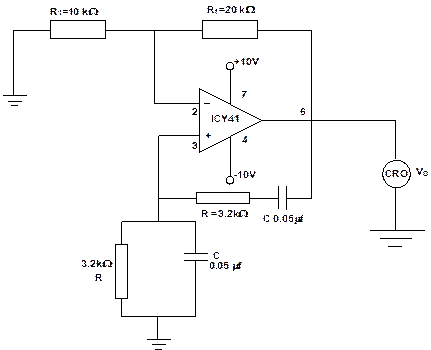Experiment No: 07

Title: Design, build and test Op Amp based Wein Bridge oscillator for given frequency.

AIM : Design, build and test Op Amp based Wein Bridge oscillator for given frequency.

APPARATUS REQUIRED:

Op-amp IC 741, Dual trace supply, Function Generator, Resistors, Capacitors, CRO, Probes

THEORY:

Equations Related to the Experiments:

a) Wein Bridge Oscillator

Closed loop gain Av = (1+Rf/R1) = 3

Frequency of Oscillation fa = 1/(2pRC)

DESIGN:

Gain required for sustained oscillation is Av = 1/b = 3

(PASS BAND GAIN) (i.e.) 1+Rf/R1 = 3

\ Rf = 2R1

Frequency of Oscillation fo = 1/2p R C

Given fo = 1 KHz

Let C = 0.05 mF

\ R = 1/2 p foC

R = 3.2 KW

Let R1 = 10 KW \ Rf = 2 * 10 KW

Circuit Diagram:Model Graph:###### PROCEDURE:
1. Connect the components as shown in the circuit Diagram
2. Switch on the power supply and CRO.
3. Note down the output voltage at CRO.
4. Plot the output waveform on the graph.
5. Redesign the circuit to generate the sine wave of frequency 2KHz.
6. Compare the output with the theoretical value of oscillation.

OBSERVATION:

Peak to peak amplitude of the output = Volts.

Frequency of oscillation = Hz.

###### Conclusion:

------------------------------------------------------------------------------------------------------------------------------------------------------------------------------------------------------------------------------------------------------------------------------------------------------------------------------------------------------------------